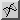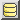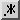Java tutorials home

 Search this site:# How do you convert to/from hexadecimal in Java?

At some point early on in your programming career you will come across hexadecimal notation. Hexadecimal (or "hex" for short) is a numbering system which works similarly to our regular decimal system, but where a single digit can take a value of 0-15 rather than 0-9. The extra digits are represented by the letters A-F, as shown in the table to the right.

00
11
...
99
10A
11B
12C
13D
14E
15F

Why use hexadecimal? Well, simply because hexadecimal is more "in tune" with various uses and instances of numbers in computing. The divisions between hexadecimal digits correspond more closely to the divisions between the bytes making up that number when it is stored. The number 1024— the multiplier for going from byte to kilobyte to megabyte etc— becomes 400 in hex and so values such as 2048 (=2K) which look a little "awkward" or "hard to judge" in decimal look simpler in hex (2048 decimal = 800 hex, for example).

So for this reason, like it or not, you will often see memory addresses, offsets, amounts of memory, bitmasks etc expressed in hex rather than decimal.

### How to convert from decimal to hex

To convert from hex to decimal in Java, assuming that you have the hex number that you want to convert stored in a String, you usually use the utility method Integer.parseString(). However, you pass 16 as the second parameter. For example:

```String hexNumber = ...
int decimal = Integer.parseInt(hexNumber, 16);
System.out.println("Hex value is " + decimal);
```

The number 16 refers to base 16, i.e. a number system (hexadecimal) where each digit represents one of 16 values (0-9 and A-F give 16 possibilities, representing the values 0-15 as in the table above).

### How to convert from decimal to hex

To go the other way round and convert decimal to hex in Java, you can use the utility method Integer.toHexString(). If you have the value that you want to convert in an int variable, then you can simply call:

```int i = ...
String hex = Integer.toHexString(i);
System.out.println("Hex value is " + hex);
```

If you have the decimal number in a String, then you first call Integer.parseInt() but this time you don't need any second parameter— decimal is the default:

```String string = ...
int no = Integer.parseInt(string);
String hex = Integer.toHexString(no);
System.out.println("Hex value is " + hex);
```

### How to convert a long to and from hex

In case you need it, the Long class has Long.parseLong() and Long.toHexString() analogous to the Integer methods above.

### How to write a hex number in Java code

Sometimes it is convenient to represent a number in hex in your Java code. There are generally two conventions for writing hex numbers. The first is to write h after the hex number. For example, 400h is the equivalent of 1024 decimal.

The second convention is to put 0x before the number, e.g. 0x400. This is the convenion used by Java:

```int noBytes = 0x400; // = 1024
```